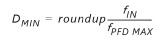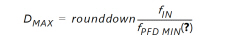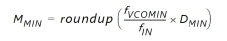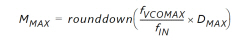# Determine the Input Frequency

## UltraScale Architecture Clocking Resources User Guide (UG572)

Document ID
UG572
Release Date
2023-02-01
Revision
1.10.2 English

The first step is to determine the input frequency. This allows all possible output frequencies to be determined by using the minimum and maximum input frequencies to define the D counter range, the VCO operating range to determine the M counter range, and the output counter range. There can be a very large number of frequencies. When using integer divides, in the worst case there are 106 x 64 x 136 = 868,363 possible combinations. In reality, the total number of different frequencies is less because the entire range of the M and D counters cannot be realized, and there is overlap between the various settings.

As an example, consider F IN = 100 MHz, F VCO = between 600 MHz and 1600 MHz, and F PFD = between 10 MHz and 550 MHz.

For a F PFDMIN of 10 MHz, the value of D can only be between 1 and 10.

D MIN (see This Equation ) = 1

D MAX (see This Equation ) = 10

For D = 1, M can only have a value between 6 and 16.

For D = 2, M can only have a value between 12 and 32.

For D = 3, M can only have a value between 24 and 64.

In addition, D = 1 M = 4 is a subset of D = 2 M = 8, D = 4 M = 16, and D = 8 M = 32 allowing these cases to be dropped. For this case, only D = 1, 3, 5, 6, 7, and 9 are considered because all other D values are subsets of these cases. This drastically reduces the number of possible output frequencies. The output frequencies are sequentially selected. The desired output frequency should be checked against the possible output frequencies generated. After the first output frequency is determined, an additional constraint can be imposed on the values of M and D. This can further limit the possible output frequencies for the second output frequency. This process is continued until all the output frequencies are selected.

The constraints used to determine the allowed M and D values are shown in these equations:

Equation 3-6Equation 3-7Equation 3-8Equation 3-9